# Number

(diff) ← Older revision | Latest revision (diff) | Newer revision → (diff)

A fundamental concept in mathematics, which has taken shape in the course of a long historical development. The origin and formulation of this concept occurred simultaneously with the birth and development of mathematics. The practical activities of mankind on the one hand, and the internal demands of mathematics on the other, have determined the development of the concept of a number.

The need to count objects led to the origin of the notion of a natural number. All nations that have forms of writing had mastered the concept of a natural number and had developed some counting system. In its early stages, the origin and development of the concept of a number can be judged only from indirect data provided by linguistics and ethnography. Primitive man clearly had no need of counting skills to determine whether or not a given collection was complete.

Later, special names were given to definite objects or events that people often encountered. Thus, in the language of certain nations there were words for such concepts as "three men" , or "three boats" , but there was no abstract concept "three" . In this way, probably, there arose comparatively short number series, used for the identification of individual people, individual boats, individual coconuts, etc. At these stage there was no such thing as an abstract number, and numbers were merely names.

In some primitive cultures, the necessity to communicate information about the numerical size of this or that collection has led to distinguishing certain standard collections, usually consisting of parts of the human body. In such a system of counting, each part of the body has a definite order and name. Whenever parts of the body are insufficient, bundles of sticks are used. The same purpose is served by pebbles, shells, notches on a tree or rock, lines on the ground, strings with knots, etc.

The next stage in the development of the notion of a number is connected with the transition to counting in groups; pairs, tens, dozens, etc. There arise so-called nodal numbers, and at the same time the concept of an arithmetic operation, which is reflected in the names of numbers. Definite counting methods take shape, special devices are used for counting, and numerical notations emerge. Numbers are separated from the objects being counted, and become abstract. Systems of representations of numbers begin to appear (cf. Numbers, representations of).

The process of formation of our modern representation system was exceptionally complicated. Only the final part of this process can be judged with definite authenticity. There are many known systems of representation. In Ancient Egypt there were several systems. In one of them there were special symbols for 1, 10, 100, 1000. Other numbers were represented by means of combinations of these symbols. The basic arithmetic operation in Ancient Egypt was addition. Well before 2000 B.C. the Babylonians used a base-60 representation system with the positional principle for writing numbers. They used only two symbols. The ancient Greeks used an alphabetic representation system, which was also used by the Slavs (cf. also Slavic numerals). In India, at the beginning of the new era (A.D.) there was a wide-spread oral positional decimal representation system, with several synonyms for zero (and other digits). A positional decimal representation system also arose there later. By the 8th century A.D. this system had spread as far as the Middle East. The Europeans were introduced to it in the 12th century.

The widening circle of objects to be counted, arising as a result of practical activities of people, and finally the inquisitiveness that is characteristic of mankind, gradually pushed back the limits of counting. The idea arose of the unbounded extension of the sequence of natural numbers, possibly attributable to the Greeks. One of Euclid's theorems states: "There exist more than any given number of primes" . Also, Archimedes tried to convince his contemporaries that it is possible to describe a number greater than "the number of grains of sand in the world" .

For the measurement of quantities, fractional numbers were necessary. Fractions were studied in Ancient Egypt and Babylon. Egyptian fractions were usually expressed in terms of aliquot fractions, i.e. fractions with numerator equal to 1. The Babylonians used base-60 fractions. The Chinese and the Indians were using ordinary fractions in the early centuries A.D., and were able to carry out all the arithmetic operations on them. Scholars in Central Asia, no later than the 10th century, used a base-60 positional counting system. This system was particularly widely used in astronomical calculations and tables. Traces of it have been passed on to us in the form of the units used in the measurement of time and angles. Decimal fractions were introduced at the beginning of the 15th century, and were widely used by the Samarkand mathematician Kashi (al'-Kashi). In Europe, decimal fractions became widespread following the publication of the book de Thiende (1585), written by S. Stevin. Before the introduction of decimal fractions, the Europeans had used the decimal system in practice to calculate the integer part of a number, but they used base-60 fractions or ordinary fractions for the fractional part.

The further development of the concept of number proceeded mainly along with the demands of mathematics itself. Negative numbers first appeared in Ancient China. Indian mathematicians discovered negative numbers while trying to formulate an algorithm for the solution of quadratic equations in all cases. Diophantus (3rd century) operated freely with negative numbers. They appear constantly in intermediate calculations in many of the problems in his Aritmetika. In the 16th century and 17th century, however, many European mathematicians did not appreciate negative numbers, and if such numbers occurred in their calculations they were referred to as false, or impossible. The situation changed in the 17th century, when a geometric interpretation of positive and negative numbers was discovered, as oppositely-directed segments.

The Babylonians had an algorithm for calculating the square root of a number to any accuracy. In the 5th century B.C., Greek mathematicians discovered that the side and diagonal of a square have no common measure. More generally, it turned out that two arbitrary, precisely-given segments are in general not commensurable. The Greek mathematicians did not start introducing new numbers. They avoided the above difficulty by creating a theory of ratios of segments that was independent of the concept of a number.

The development of algebra, and the techniques of approximate calculation, in connection with the demands of astronomy, led Arab mathematicians to extend the concept of a number. They began to consider ratios of arbitrary quantities, whether commensurable or not, as numbers. As Nasireddin (1201–1278) wrote: "Each of these ratios can be called by a number, precisely equal to the number one when one term of the ratio agrees with the other term" . European mathematics was developing in the same direction. Although G. Cardano in Practica Arithmeticae Generalis (1539) was still writing about irrational numbers as "surds" (from the Latin "surdus" , "deaf" ), and as "impossible to perceive or to imagine" , Stevin in his l'Arithmétique (1585) stated that "a number is that which is determined by an arbitrary quantity" and that "no numbers are absurd, irrational, irregular, inexpressible, or surd" . And finally I. Newton in his Arithmeticae Universalis (1707) gave the following definition: "By a number we understand not so much a multiple of a unit as an abstract quantity associated in a systematic way to some other quantity of the same kind that is taken as a unit. Numbers arise in three forms: integer, fraction and irrational. An integer is that which can be measured by unity; a fraction is a multiple of a portion of unity; an irrational number is incommensurable with unity" . Related to the fact that, for Newton, a quantity can be either positive or negative, the numbers in his arithmetic can also be either positive, in other words "greater than nothing" , or negative, in other words "smaller than nothing" .

Imaginary numbers first appeared in the work of Cardano, Ars Magma (The Great Art, 1545). In solving the system of equations,, he found the solutions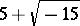and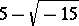. Cardano called these solutions "purely negative" , and later "sophisticatedly negative" . The first to see a "real" use of introducing imaginary numbers was R. Bombelli. In his Algebra (1572) he showed that the real roots of the equation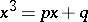,,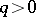, in the case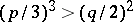, can be expressed in terms of radicals of imaginary numbers. Bombelli defined arithmetic operations on such quantities, and proceeded in this way to the creation of the theory of complex numbers (cf. Complex number). In the 17th century and 18th century, many mathematicians occupied themselves in investigating the properties of imaginary numbers and their applications. Thus, L. Euler extended, e.g., the notion of a logarithm to arbitrary complex numbers (1738), and obtained a new method of integration using complex variables (1776), while earlier (1736) A. de Moivre solved the problem of extracting roots of natural degrees of an arbitrary complex number. A successful application of the theory of complex numbers was the fundamental theorem of algebra: "Every polynomial of degree greater than zero and with real coefficients factorizes as a product of polynomials of degrees one and two with real coefficients" (Euler, J. d'Alembert, C.F. Gauss). Nevertheless, until a geometric interpretation of complex numbers as points in the plane was given (around the end of the 18th century and in the beginning of the 19th century), many mathematicians remained distrustful of imaginary numbers.

In the early 19th century, in connection with the great successes of mathematical analysis, many scholars realized the need for a foundation of the fundamentals of analysis — the theory of limits. Mathematicians were no longer satisfied with proofs based on intuition or on geometrical representation. There also remained the problem of constructing a unified theory of numbers. Natural numbers were often thought of as collections of unity, fractions as ratios of quantities, real numbers as lengths of line segments, and complex numbers as points in the plane. There was no complete agreement as to how arithmetic operations on numbers should be introduced. Finally, the question naturally arose of the further development of the concept of a number. In particular, was it possible to introduce new numbers, related to points in space?

The 19th century saw intensive research in all the above directions. A general principle was formulated according to which any generalization of the concept of number should proceed — the so-called principle of permanence of formal computing laws. According to this, when constructing a new number system extending a given system, the operations should generalize in such a way that the existing laws remain in force (G. Peacock, 1834; H. Hankel, 1867). In the second half of the 19th century, the theory of real numbers (cf. Real number) was constructed almost simultaneously by G. Cantor (1879), Ch. Meray (1869), R. Dedekind (1872), and K. Weierstrass (1872). Here Cantor and Meray used fundamental sequences of rational numbers, Dedekind used cuts in the field of rational numbers, and Weierstrass used infinite decimal expansions.

As a result of the work of G. Peano (1891), Weierstrass (1878) and H. Grassmann (1861), an axiomatic theory of natural numbers was constructed. W. Hamilton (1837) constructed a theory of complex numbers from pairs of real numbers, Weierstrass constructed a theory of integers from pairs of natural numbers, and J. Tannery (1894) constructed a theory of rational numbers from pairs of integers.

Attempts to find generalizations of the concept of a complex number led to the theory of hypercomplex numbers (cf. Hypercomplex number). Historically, the first such number system was the quaternions (cf. Quaternion), discovered by Hamilton. After much investigation it became clear (Weierstrass, G. Frobenius, B. Pierce) that any extension of the concept of a complex number beyond the system of complex numbers itself is possible only at the cost of some of the usual properties of numbers.

Throughout the 19th century, and into the early 20th century, deep changes were taking place in mathematics. Conceptions about the objects and the aims of mathematics were changing. The axiomatic method of constructing mathematics on set-theoretic foundations was gradually taking shape. In this context, every mathematical theory is the study of some algebraic system. In other words, it is the study of a set with distinguished relations, in particular algebraic operations, satisfying some predetermined conditions, or axioms.

From this point of view every number system is an algebraic system. For the definition of concrete number systems it is convenient to use the notion of an "extension of an algebraic system" . This notion makes precise in a natural way the principle of permanence of formal computing laws, which was formulated above. An algebraic system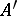is called an extension of an algebraic systemif the underlying set ofis a subset of that of, if there also exists a bijection from the set of relations of the systemonto that of, and if for any collection of elements of the systemfor which some relation of that system holds, the corresponding relation of the systemalso holds.

For example, by the system of natural numbers one usually understands the algebraic systemwith two algebraic operations: addition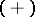and multiplication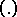, and a distinguished element

(unity), satisfying the following axioms:

1) for each element,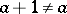;

2) associativity of addition: For any elements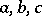in,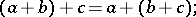3) commutativity of addition: For any elements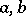in,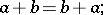4) cancellation of addition: For any elementsin, the equationentails the equation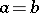;

5) 1 is the neutral element for the multiplication; that is, for anyone has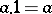;

6) associativity of multiplication: For any elementsin,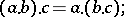7) distributivity of multiplication over addition: For any elementsin,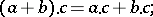8) the axiom of induction: Ifis a subset ofcontaining 1 and the elementwhenever it contains, then.

From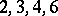, andit follows that the system of natural numbers is a semi-ring under the operationsand. Hence the system of natural numbers can be defined as the minimal semi-ring with a neutral element for multiplication and without a neutral element for the addition.

The system of integers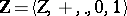is defined as the minimal ring that is an extension of the semi-ring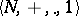of natural numbers. The system of rational numbers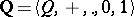is defined as the minimal field that is an extension of the ring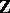. The system of complex numbers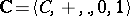is defined as the minimal field that is an extension of the fieldof real numbers containing an elementfor which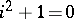(cf. also Extension of a field).

By the system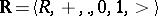of real numbers one means the algebraic system with two binary operationsand, two distinguished elements

and

and binary order relation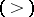. The axioms ofare divided into the following groups:

1) the field axiom: The system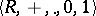is a field;

2) the order axiom: The system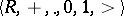is a totally and strictly ordered field (cf. Ordered field);

3) the Archimedean axiom: For any elements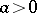,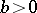inthere exists a natural numbersuch that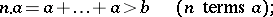4) the completeness axiom: Every fundamental sequenceof real numbers converges, i.e. if for anythere is a number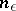such that, for any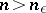and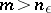the inequality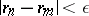holds, then the sequence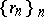converges to some element of.

Briefly, the system of real numbers is a complete, totally, strictly-Archimedean ordered field. The system of real numbers can also be defined, in an equivalent way, as a continuous totally ordered field. In this case the Archimedean axiom and the completeness axiom are replaced by the continuity axiom:

Ifandare non-empty subsets ofsuch that, for any elements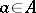,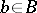, the inequalityholds, then there exists an element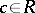such thatfor all,.

The construction of real numbers proposed by Cantor and Meray can be used to interpret the first system of axioms for the system of real numbers, while Dedekind's construction can be used to interpret the second system. Analogously, the constructions of Hamilton, Weierstrass and Tannery are interpretations of the systems of axioms for the complex, integer and rational numbers.

As interpretations of the system of natural numbers one may use the ordinal theory of natural numbers developed by Peano, and the cardinal theory of natural numbers of Cantor.

The problem of the foundations of the concept of a number, and more broadly, the foundations of mathematics, were clearly set out in the 19th century. This problem became a subject of mathematical logic, the intensive development of which continued into the 20th century.

How to Cite This Entry:
Number. Encyclopedia of Mathematics. URL: http://encyclopediaofmath.org/index.php?title=Number&oldid=11869
This article was adapted from an original article by V.I. Nechaev (originator), which appeared in Encyclopedia of Mathematics - ISBN 1402006098. See original article